Previous: 4.5 Initial Values and Up: 4. Tuning of the Next: 4.7 Comparison with Monte

# 4.6 Variations of the Newton Method

For a fixed potential we can solve the set of moment equations reliably. The Newton method is robust in this case. However, convergence problems emerge when we couple the Boltzmann moment system with the Poisson equation.

In principle it is possible to eliminate the potential from the system by solving the Poisson equation for the electrical field on the fly'', which makes it a function of the density. This approach was tried, but it degraded the performance of the nonlinear solver. The other extreme is to solve for the moments using a fixed potential. In this case the potential is the primary variable. Interpolating approaches are possible and are studied below.

The first variant which was tried was dubbed residual damping''. It consists in using a modified residuum vector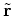in the Newton equation(4.8)

Now the components of the residuum vector (from the potential and the even moments) are rescaled by factors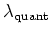, e.g., in the form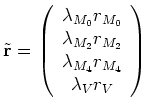(4.9)

We mostly used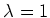for the even moments and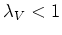. This corresponds to changing the potential slowly with the even moments closer to a non-selfconsistent solution.

An alternative implementation of a similar idea is to change the Jacobian by adding a diagonal matrix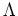, as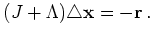(4.10)

This assumes that the Jacobian has previously been rescaled to unit diagonal in a first step and is diagonal dominant.

The diagonal entries inare chosen block-wise according to the type of quantity. This method has nice mathematical properties. For the diagonal matrixwe recover the original equation. Letting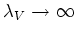(and zeroes for the other blocks) gives the moment system for a fixed potential in the limit.

The implementation in MINIMOS-NT takes another, more sophisticated approach. Here the Jacobian is calculated symbolically. Parts of the Jacobian are initially neglected and only switched on'' later in the Newton iteration. With this method convergence can often be obtained without the need for bias stepping.